# Need help with Simple Flexible beam and dynamic model of mechanical part

Hello, i am new to ansys and dont have much idea about this software, i just used it to calculate the harmonic response of simplest beam by watching the youtube video and i thought that's it what i need to continue my work in matlab. but now my teacher said something which i don't understand. because i dont know the how deep is the ansys work due to lack of knowledge about this software. please have a look and help me explain what my teacher is asking for.

My topic: control of Flexible manipulator (SDOF)

i made mathematical model, made simplest geometry in ansys, and got the harmonic response so i could use the natural frequency as parameter value for the controller which i need to make in MATLAB.

then my teacher asked me to link up my ansys analysis to the system parameters. (How? what parameters is he talking about) i asked him is he talking about natural frequency, inertia, deformation!

and this is what he replied instead of clear answer "Some of the parameters must be obtained from Ansys analysis.  You will have to show us the process. How did you get the dynamic model of the mechanical part? What's the use of the Ansys analysis if you can not use it to derive your dynamic model?""

after reading this reply i am more confuse than ever. can anyone tell me what kind of parameters is he talking about?

look at the picture, simplest possible small beam with no extra complication. so what parameters could he be talking about?

• Here is your image for the ANSYS staff who are not permitted to open attachments.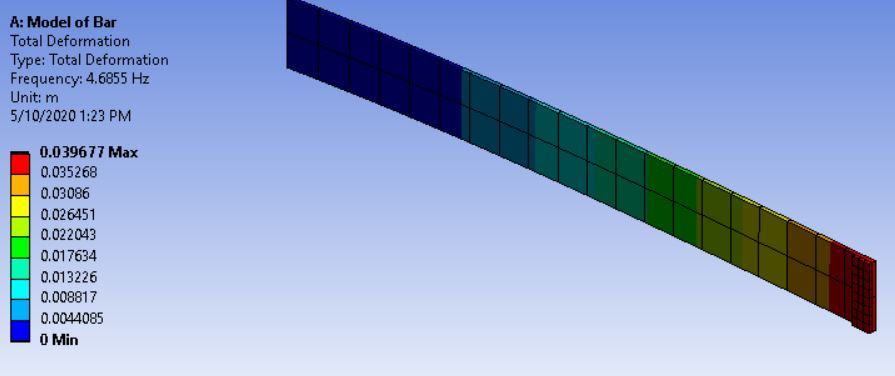• Please reply with a system block diagram of the control system.

What are the inputs to the flexible manipulator?

What are the outputs from the flexible manipulator?

• Hello, thank you for your reply. there is limit of words therefore 3 messages. pardon.here is the link of the model i am trying to make virtually. it will give you better idea what i want.

but in this video there is no extra point mass at the tip of the ruler(non-fixed end). in my geometry i need extra point mass so i can get the response of deformation.

Difficulties i am having as a new user:

To find stiffness of the beam (linear and angular) - the videos i found on internet have 2 objects and then they attach spring to find stiffness but in my case its already a beam so couldn't find any tutorial to follow.

To find moment of inertia of the beam and point mass - i can see the moment of inertia in the "details" but can find the inertia only when i select the geometry of specific part. this value is for single object as i need the moment of inertia required for point mass as well because the combined inertia will be required to rotate the beam.

• Reverse setup: 2 things i need to calculate to select a motor for the beam to rotate. 1st: i need to calculate how much torque is required to move the beam from initial position to 30degree angle or 25degree. which i am unable to do. which setting will get me the torque required? 2nd: when i was trying to find the force required to move the beam to certain distance from initial position to lets say 0.15m, i found a video which showed how we can get that by using the reaction forces but required stiffness value which i don't have. how can i get these things?

Adding point mass: need suggestion for this confusion: when i just selected the face and added point mass, it was just point mass, i couldn't apply any forces or other features on it. then i tried to add another geometry like it is shown in the picture of my post you posted as well. at the end i just added a small block and gave it the mass of 0.5kg. then total weight became 1kg. so my question here is, should i add another object like shown in the picture above? or should i use just point mass if i want to get the effect of point mass? please suggest.  and will the moment of inertia of both masses will be combined or not? if not then how to combine or get the exact value required to rotate the beam horizontally.

Thanks.

• you asked for system block diagram of control system? are you asking for matlab model? simulink?

inputs of flexible manipulator? probably torque. or are you asking for the state-space?

outputs of the flexible manipulator? position and deflection angle.

if those doesn't cover your answer i can provide the matrices or other details if that can be helpful. thanks again

I just tried random things like remote displacement, randomly added point mass, random value force just to check the changes. but i have no idea how to precisely calculate these things so it will be good enough to write it in my report with results. here is the sample picture done in a random manner. one more question if you check the moment of inertia detail part you will see there are 3 tabs lp1,lp2,lp3,what is the meaning of lp1,lp2,lp3. Is this some kind of axis indication? Does lp1 mean x-axis? Or something like this? Please find sometime to answer..i will be grateful.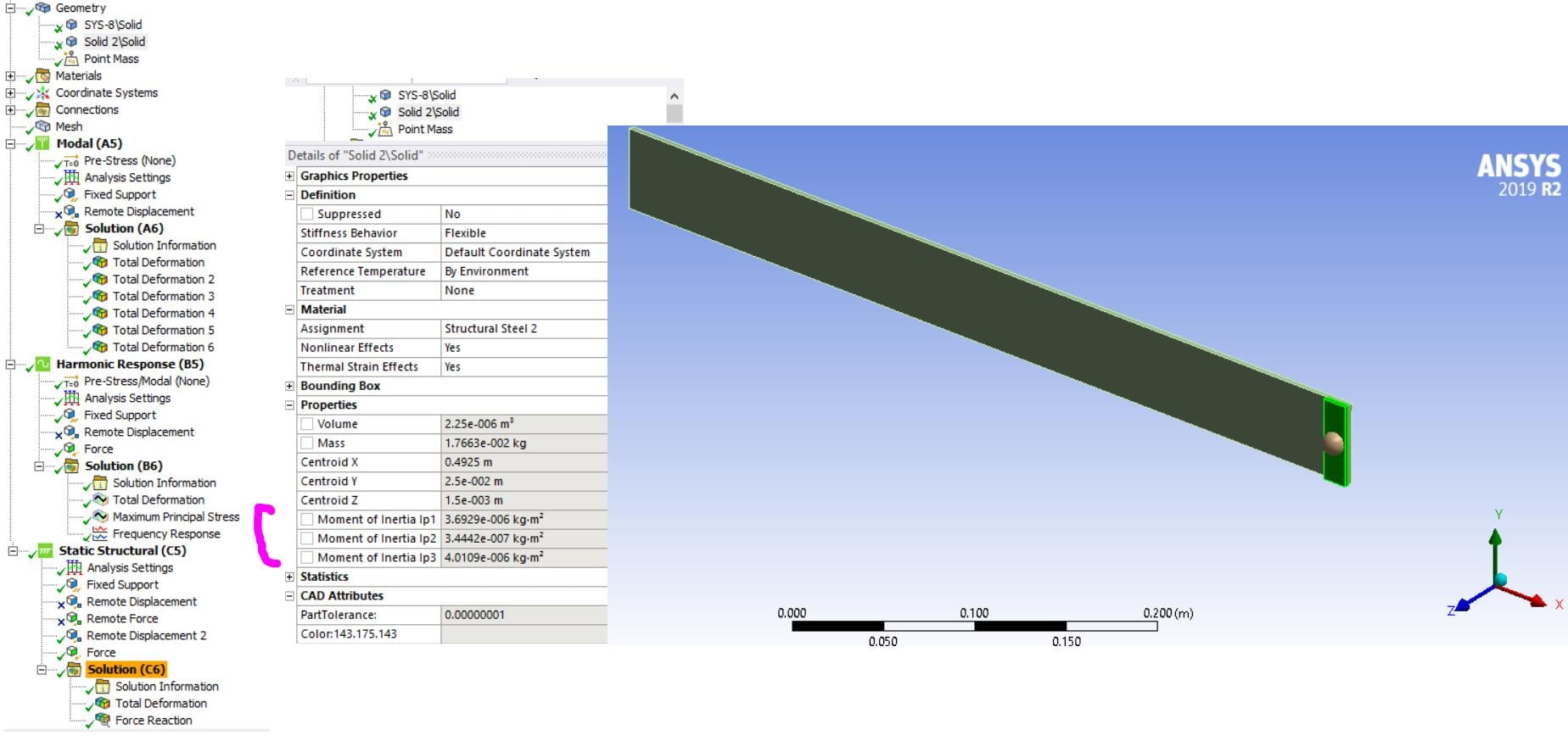• Thank you for the video, that is very interesting.

Stiffness is a property that can be measured between two points. What two points do you want to use to define stiffness? Stiffness is also directional. A beam has different stiffness for deformation in the X, Y and Z directions as well as torsional stiffness about Rx, Ry and Rz. You have to define stiffness more clearly.

You show a solid plate for the flexible beam. Don't use a solid, use a surface body, mesh it with shell elements and assign them a thickness property.

Here is a 0.25 kg Point Mass on the end of a 0.0314 kg cantilever beam 200 x 20 x 1 mm.  The point mass is scoped to the short edge of the cantilever.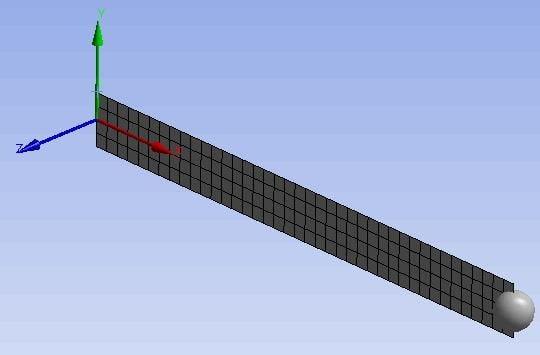Note that this is a true point mass. The Moments of Inertia are zero. To simulate a body that spans the width of the cantilever like you did, you could take the values of Ip from that body and type them into the details of the point mass.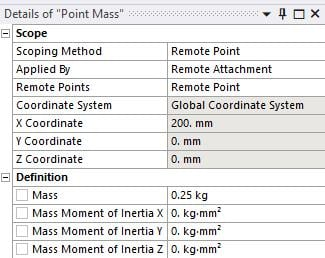Make the edge on the Y axis Fixed and run a Modal Analysis. Click on the Solution Information folder and look at the Solver Output. In that listing you will find the Precise Mass Summary with the Moment of Inertia about the Origin. You want to use the values the green arrow points to.  You don't want the values the red arrow points to because they are not where the torque is applied.  Note that the units are very important!  These units are kg and m.  Be careful, since you will get different numbers if the units are set to mm.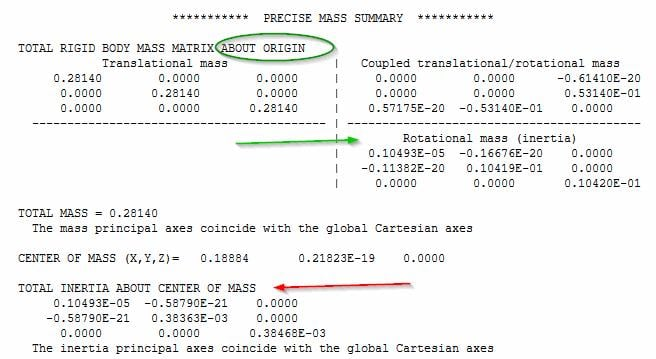• Thank you very much for the reply. highly appreciated. the model you have provided is kind of perfect like i want. thank you again

Stiffness is directional: The beam is required to move horizontally (Z-axis/direction) as shown in video.

A beam has different stiffness for deformation in the X, Y and Z directions as well as torsional stiffness about Rx, Ry and Rz: Torsional stiffness shows how much resistance it offers per degree change in its angle when twisted. but we are not twisting the beam. or are we? will it be considered as twisting in flexible state?

Define Two point for stiffness-i am not sure/i am confuse. one fixed end, one non-fixed end, i want to move the beam to 30 degree angle from its initial zero position. so i guess the points will be fixed and non-fixed end! if i am wrong please correct me. the way i understand is that "when we apply the controlled motor torque to rotate the beam to specific angle or distance, then beam itself have some oscillations depending on stiffness" so wont we check the overall stiffness according to the behavior or the beam? or do we just simply calculate angular/linear stiffness with specific points?

i still don't understand that what is the meaning/use of lp1,lp2,lp3 in inertia section.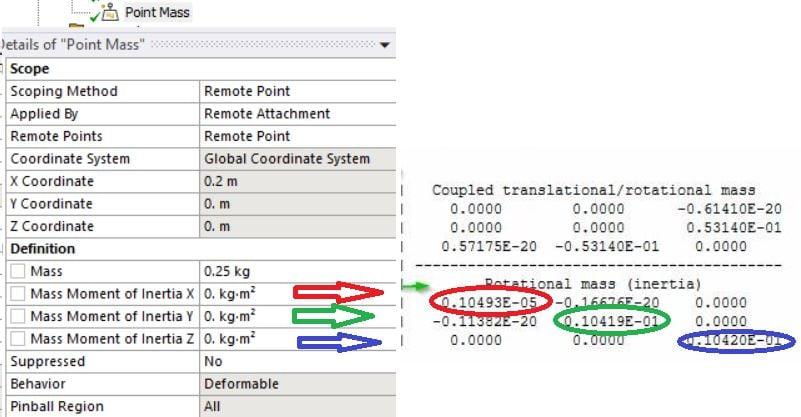is this how i am suppose to fill the values...right?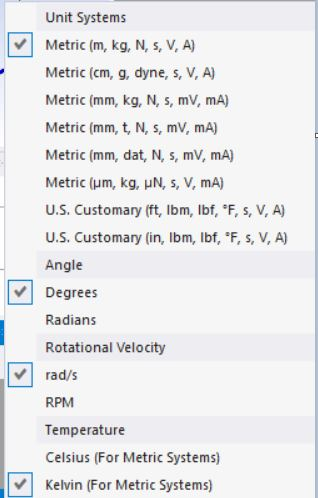and as you said the units are important. i am not getting the same values as yours even after changing to m, kg unit standards. changing the standards are not effecting, so it doesn't matter which standard i choose, its changing the value in the properties box but not in the "solution information"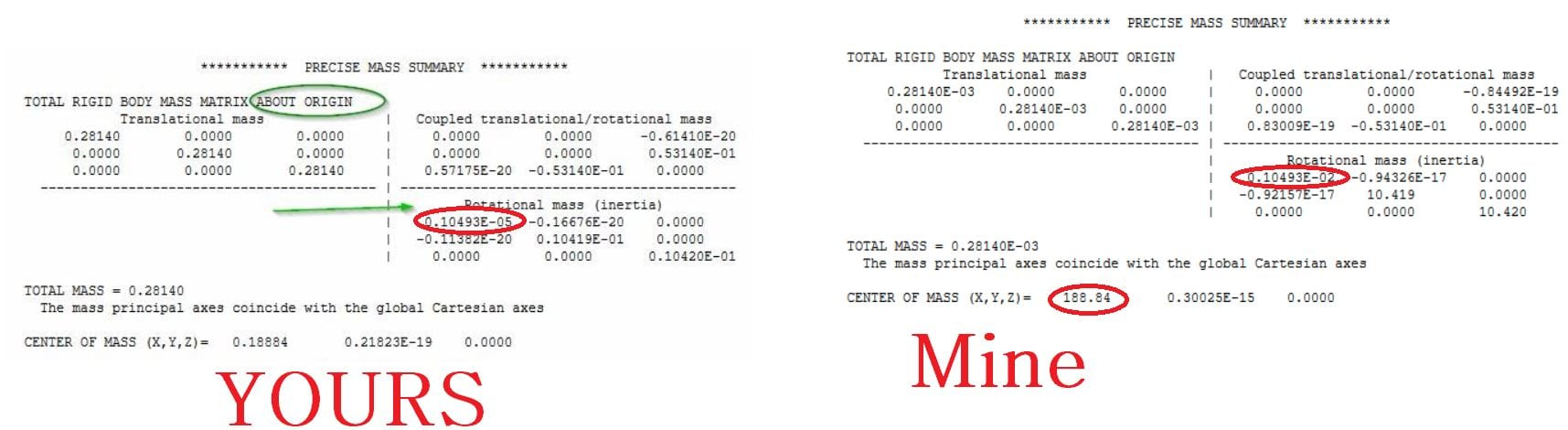although its just the conversion issue but still i would like to solve it. thanks

• YOURS vs Mine
In YOURS, the units are set to mm, in Mine, the units were set to SI. You can verify that by looking higher up in the Output. You have to Clear Generated Data after you change to SI units before you Solve, otherwise, Workbench sees the model is already solved and will not solve it again. Below is what you had in the output listing.

` MPA UNITS SPECIFIED FOR INTERNAL      LENGTH      = MILLIMETERS (mm)  MASS        = TONNE (Mg)  TIME        = SECONDS (sec)  FORCE       = NEWTON (N)`

Beam Stiffness in different directions
If I define the two points for stiffness as the ends of the cantilever, I can apply a Z displacement or I can apply a Z rotation at the tip.  The resulting deformation in the middle is different and the stiffness values aren't even in the same units, one is in N/m and the other is in N-m/degree. In the image below, I have overlaid two cantilevers and applied a Z displacement to one and a Z rotation to the other.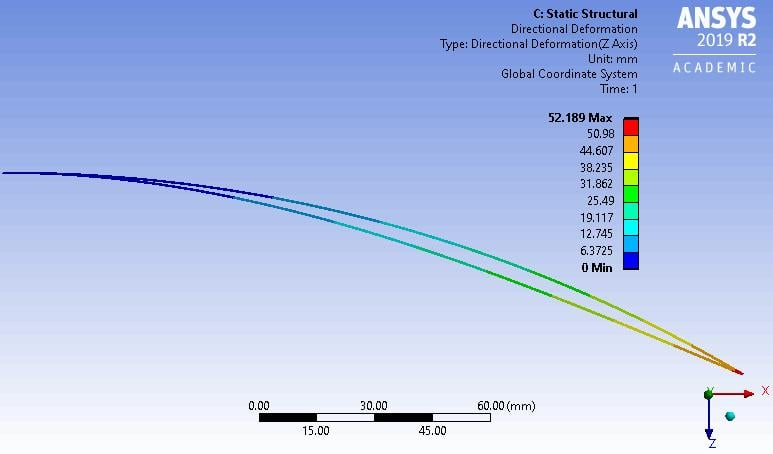You are confusing a Rigid Body Rotation of 30 degrees at the base, which if done slowly, causes no deformation and results in a perfectly straight cantilever... with applying a 30 degree static rotation of the tip relative to a fixed base that cause the deformation shown above.  In your dynamic case, the inertia of the mass at the tip and the rapid rotation of the shaft connected to the base of the cantilever results in deformation similar to that shown above.

Moments of Inertia
You should read a textbook to understand mass moments of inertia. A solid body has a 3x3 matrix for the mass moments of inertia relative to a specific coordinate system, such as the one at the base of the cantilever.  This can have non-zero off-diagonal terms in the matrix.  Then there is a special moment of inertia matrix, relative to a coordinate system at the centroid of the mass and oriented in a special way such that the moments of inertia values are only on the diagonal and all the off-diagonal terms are zero. Those three values are called the Principal moments of inertia.

• thank you...i will look into it. did you happen to save the file when you performed this static structural directional deformation? can you share? thanks.

the beam will be same as before without deformation if done slowly, but i have to do it in less time like 0.09 sec or 0.1sec etc...so considering the thickness it will definitely produce deformation.

• may be i am missing something, i tried but couldn't bend the beam the way you have done it, its not bending much, can you please share the file you made? so i can check what is i am missing... or the other way around, i am attaching the file, may be you can have a look. i might be doing this the wrong way. if you do not have the time to look at it, then please share yours. thanks

• Open SpaceClaim, on the Prepare tab, use the Midsurface button to replace the solid body with a sheet body.

In Mechanical, reattach the Fixed Support and Remote Displacement to the edges of the sheet body.

Under the Static Structural analysis, under the Analysis Settings, turn on Large Deflection.

Solve it once with a 30 degree rotation about the Z axis, leaving the Z displacement free. Note the directional displacement in the Z direction.

Solve it again with the Z rotation free and apply the Z displacement found in the previous solution. Those are the two curves.

If you want the two curves overlaid, then copy and paste the midsurface so you have two cantilever beams in the same model, but give them the two different end conditions.  If you do that, you will need to increase the nonlinear displacement convergence tolerance to make the tips end up at the same value.

• Thank you very much for reply in details. i tried and almost everything worked.

few things: when i am trying to add displacement its not rotating the bar as i want, may be some bug but if add y-axis rotation it rotates in z-axis and gives the result

before you said The resulting deformation in the middle is different and the stiffness values aren't even in the same units, one is in N/m and the other is in N-m/degree. where are you checking it. i cant seem to find the value or unit mentioned anywhere.

i was trying different things and selected the beam probe and found many options,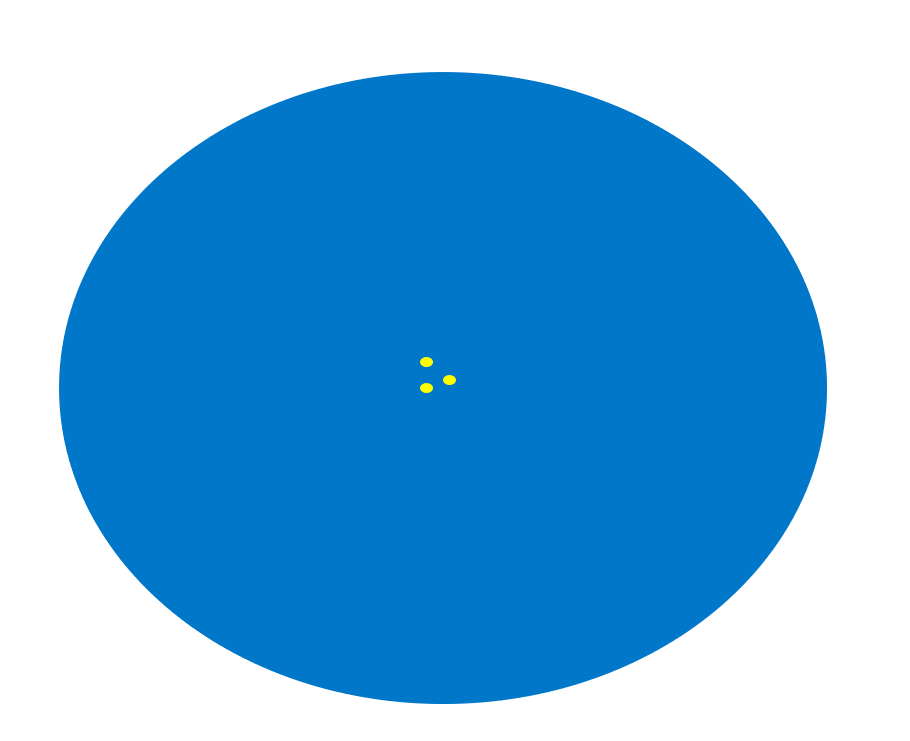• Use a Remote Displacement on the tip of the cantilever. There you can apply a 30 degree rotation.  The base of the cantilever can also have a remote displacement with all six DOF set to 0.

Insert into the solution a Probe > Reaction Moment > and choose the Remote Displacement.  The probe will tell you how many N-m of torque is on the cantilever, the Remote Displacement has the 30 degree input. So divide the Torque by 30 to get N-m/degree.